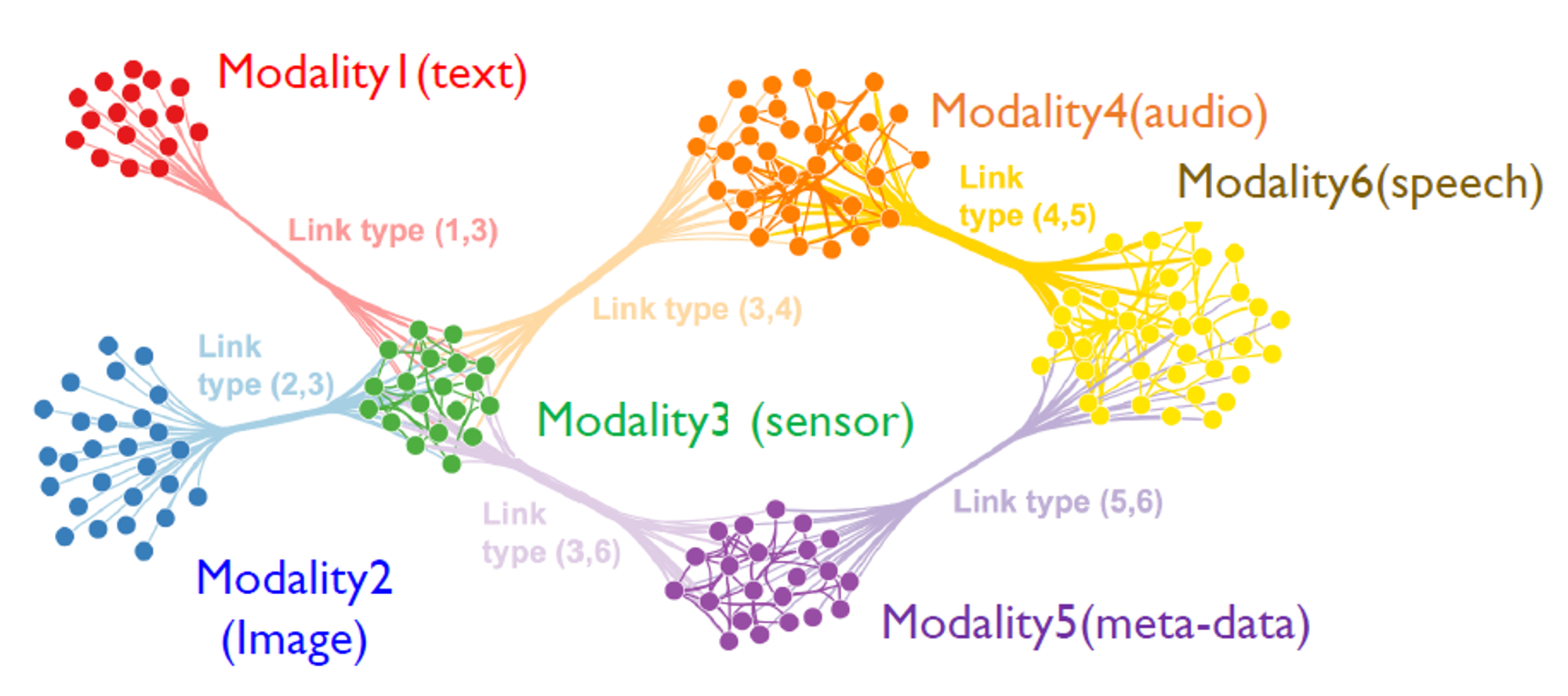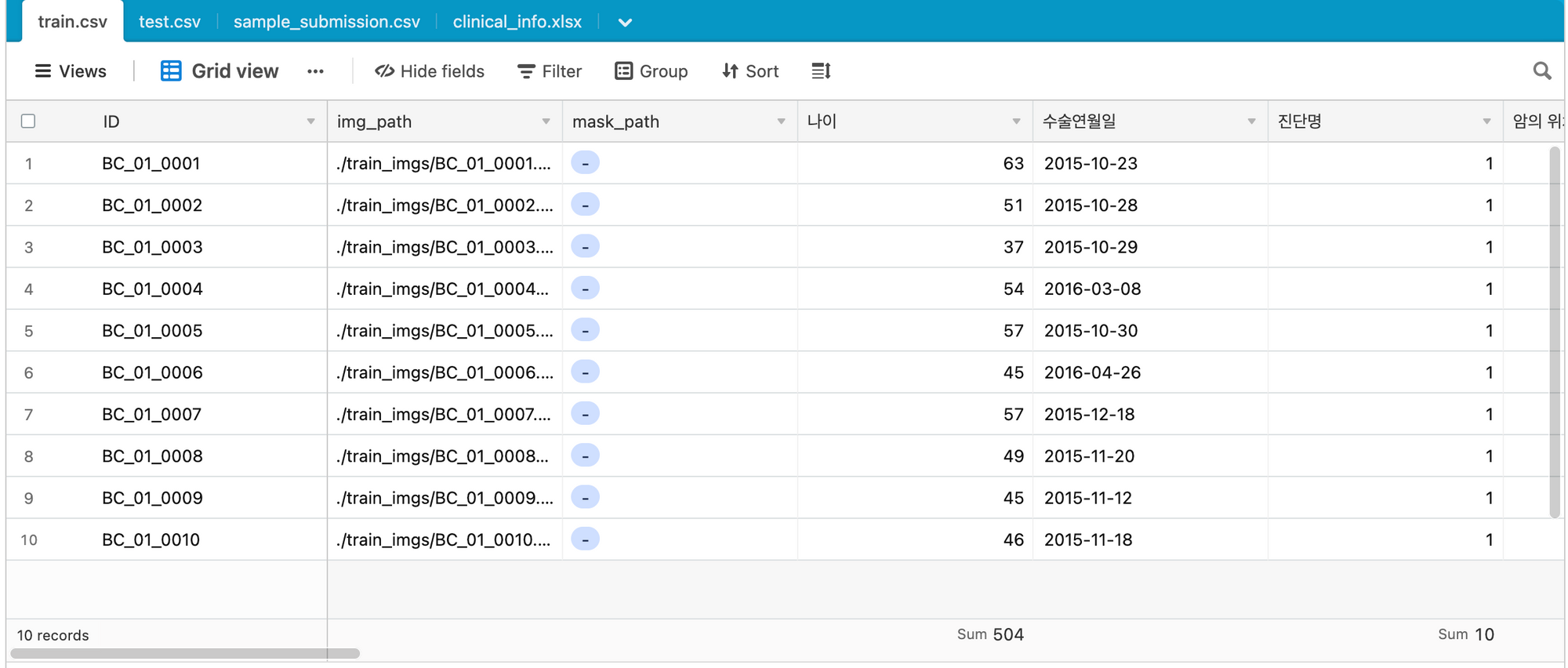# Code Review : Baseline Code for AI competition for predicting lymphadenopathy in breast cancer

aerojohn1223·2023년 4월 29일
0

목록 보기
1/2

## Multi-Modal Learning

Training the Model with datas of various modality that is collected by 5 senses of human, which are 1) Vision, 2) Text, 3) Speech, 4) Touch, 5) Smell, +) Meta Data.The model is trained by data with different feature dimensions simultaneously.

To learn well, the data with different feature dimensions must be integrated well.

There are 3 ways of integrating datas of different feature dimensions.

1) Data-level integration

2) Classifier integration

3) Embedding Vector integration

The code that is reviewed here uses the third way of integration.

## Competition and Baseline Code

Competition Baseline Code

### DataImage Data + (Categorical/Numerical Data)

### Import

import random
import pandas as pd
import numpy as np
import os
import cv2
from tqdm.auto import tqdm

import torch
import torch.nn as nn
import torch.optim as optim
import torch.nn.functional as F

import albumentations as A
from albumentations.pytorch.transforms import ToTensorV2

import torchvision.models as models

from sklearn import metrics
from sklearn.preprocessing import StandardScaler, LabelEncoder

from sklearn.model_selection import train_test_split

import warnings
warnings.filterwarnings(action='ignore')

torch : OpenSource Framework for Machine Learning

sklearn(scikit learn) : OpenSource Machine Learning Library

albumentations : Library for augmentations

device = torch.device('cuda') if torch.cuda.is_available() else torch.device('cpu')

set the device to GPU(cuda)

### Hyperparameter Setting

CFG = {
'IMG_SIZE':512,
'EPOCHS':5,
'LEARNING_RATE':1e-4,
'BATCH_SIZE':16,
'SEED':41
}

### Fixed RandomSeed

def seed_everything(seed):
random.seed(seed)
os.environ['PYTHONHASHSEED'] = str(seed)
np.random.seed(seed)
torch.manual_seed(seed)
torch.cuda.manual_seed(seed)
torch.backends.cudnn.deterministic = True
torch.backends.cudnn.benchmark = True

seed_everything(CFG['SEED']) # Seed 고정

### Data Preprocessing

#Load DataFrame

test_df = pd.read_csv('./test.csv')
#Fill Null

train_df['암의 장경'] = train_df['암의 장경'].fillna(train_df['암의 장경'].mean())
train_df = train_df.fillna(0)

test_df['암의 장경'] = test_df['암의 장경'].fillna(train_df['암의 장경'].mean())
test_df = test_df.fillna(0)

1) For the column “암의 장경”, the longest diameter of the cancer, the null values were filled by the mean of the column.

2) For the rest of the columns, the null values were filled with 0.

#Train, Validation Split

train_df, val_df, train_labels, val_labels = train_test_split(
train_df.drop(columns=['N_category']),
train_df['N_category'],
test_size=0.2,
random_state=CFG['SEED']
)

train_df is separated to train_df and val_df. train_labels and val_labels were also separated from the train_df and val_df.

#Numerical Feature Scaling / Categorical Feature Label-Encoding

def get_values(value):
return value.values.reshape(-1, 1)

numeric_cols = ['나이', '암의 장경', 'ER_Allred_score', 'PR_Allred_score',
'KI-67_LI_percent', 'HER2_SISH_ratio']
ignore_cols = ['ID', 'img_path', 'mask_path', '수술연월일', 'N_category']

for col in train_df.columns:
if col in ignore_cols: #for columns that are not numerical or categorical,
continue           #continue
if col in numeric_cols: #Numerical Columns
scaler = StandardScaler()
train_df[col] = scaler.fit_transform(get_values(train_df[col]))
val_df[col] = scaler.transform(get_values(val_df[col]))
test_df[col] = scaler.transform(get_values(test_df[col]))
else: #Categorical Columns
le = LabelEncoder()
train_df[col] = le.fit_transform(get_values(train_df[col]))
val_df[col] = le.transform(get_values(val_df[col]))
test_df[col] = le.transform(get_values(test_df[col]))

get_values( ) : changes DataFrame into an array, so that scaling or label encoding can be done.

numeric_cols: list of numerical columns

ignore_cols : list of columns that are not numerical or categorical.

StandardScaler( ) : When analyzing multiple units, Standardization or normalization should be done. StandardScaler is a function for normalization, which means all the values are normalized with a mean of 0 and a variance of 1.

LabelEncoder( ) : LabelEncoder is a function that changes categorical data to numerical data. For example, if there are ‘apple’, ‘banana’, ‘orange’ in the column, all ‘apple’ data will become 1, all ‘banana’ data will become 2, and all ‘orange’ data will become 3.

### Custom Dataset

class CustomDataset(Dataset):
def __init__(self, medical_df, labels, transforms=None):
self.medical_df = medical_df
self.transforms = transforms
self.labels = labels

def __getitem__(self, index):
img_path = self.medical_df['img_path'].iloc[index]
image = cv2.cvtColor(image, cv2.COLOR_BGR2RGB)

if self.transforms is not None:
image = self.transforms(image=image)['image']

if self.labels is not None:
tabular = torch.Tensor(self.medical_df.drop(columns=['ID', 'img_path', 'mask_path', '수술연월일']).iloc[index])
label = self.labels[index]
return image, tabular, label
else:
tabular = torch.Tensor(self.medical_df.drop(columns=['ID', 'img_path', '수술연월일']).iloc[index])
return image, tabular

def __len__(self):
return len(self.medical_df)

3 functions are defined within the class CustomDataset for pytorch.

1) init : declares needed variables

1) getitem : reads image data, and creates tabular data and label data. Also returns them.

2) len : returns the length of the dataframe.

cv2.Color(image, cv2.COLOR_BGR2RGB) : when an image is imported by opencv, the image is save in the order of BGR. In order to look at the image correctly, we have to change the order to RGB.

For the train set and validation set, categorical columns and numerical columns are stored in the variable ‘tabular’. Labels are stored in the variable ‘label’.

For the test set, categorical columns and numerical columns are stored in the variable ‘tabular’.

train_transforms = A.Compose([
A.HorizontalFlip(),
A.VerticalFlip(),
A.Rotate(limit=90, border_mode=cv2.BORDER_CONSTANT,p=0.3),
A.Resize(CFG['IMG_SIZE'],CFG['IMG_SIZE']),
A.Normalize(mean=(0.485, 0.456, 0.406), std=(0.229, 0.224, 0.225), max_pixel_value=255.0, always_apply=False, p=1.0),
ToTensorV2()
])

test_transforms = A.Compose([
A.Resize(CFG['IMG_SIZE'],CFG['IMG_SIZE']),
A.Normalize(mean=(0.485, 0.456, 0.406), std=(0.229, 0.224, 0.225), max_pixel_value=255.0, always_apply=False, p=1.0),
ToTensorV2()
])

Albumentations: Python library for image augmentation.

Functions and Hyperparameters of Albumentations:

Rotate(limit = n, border_mode= m ) : Rotates the image. limit is the hyperparameter that limits the image to be rotated within -n to n. You can choose how you will deal with the leftover space with hyoperparameter border_mode. You can also choose the probability of the augmentation with hyperparameter p.

Resize(height, weight) : changes the size of the image.

Normalize(mean = ( ), std = ( ), max_pixel_value = y) : Image normalization. Normalization is done by the following formula.

img = (img - mean * max_pixel_value) / (std * max_pixel_value)

ToTensorV2 : Converts images to tensors

train_dataset = CustomDataset(train_df, train_labels.values, train_transforms)

val_dataset = CustomDataset(val_df, val_labels.values, test_transforms)
val_loader = DataLoader(val_dataset, batch_size=CFG['BATCH_SIZE'], shuffle=False, num_workers=0)

### Model Architecture

class ImgFeatureExtractor(nn.Module):
def __init__(self):
super(ImgFeatureExtractor, self).__init__()
self.backbone = models.efficientnet_b0(pretrained=True)
self.embedding = nn.Linear(1000,512)

def forward(self, x):
x = self.backbone(x)
x = self.embedding(x)
return x

ImgFeatureExtractor : Extracts the features of image datas.

Pretrained effienctnet_b0 was used for ImgFeatureExtractor.

class TabularFeatureExtractor(nn.Module):
def __init__(self):
super(TabularFeatureExtractor, self).__init__()
self.embedding = nn.Sequential(
nn.Linear(in_features=23, out_features=128),
nn.BatchNorm1d(128),
nn.LeakyReLU(),
nn.Linear(in_features=128, out_features=256),
nn.BatchNorm1d(256),
nn.LeakyReLU(),
nn.Linear(in_features=256, out_features=512),
nn.BatchNorm1d(512),
nn.LeakyReLU(),
nn.Linear(in_features=512, out_features=512)
)

def forward(self, x):
x = self.embedding(x)
return x

TabularFeatureExtractor : Extracts the features from the datas that are stored in variable ‘tabular’.

class ClassificationModel(nn.Module):
def __init__(self):
super(ClassificationModel, self).__init__()
self.img_feature_extractor = ImgFeatureExtractor()
self.tabular_feature_extractor = TabularFeatureExtractor()
self.classifier = nn.Sequential(
nn.Linear(in_features=1024, out_features=1),
nn.Sigmoid(),
)

def forward(self, img, tabular):
img_feature = self.img_feature_extractor(img)
tabular_feature = self.tabular_feature_extractor(tabular)
feature = torch.cat([img_feature, tabular_feature], dim=-1)
output = self.classifier(feature)
return output

With the features extracted by ImgFeatureExtractor and TabularFeatureExtractor, ClassificationModel classifies the images.

### Train

def train(model, optimizer, train_loader, val_loader, scheduler, device):
model.to(device)
criterion = nn.BCEWithLogitsLoss().to(device)#Sigmoid Layer + BCELoss

best_score = 0
best_model = None

for epoch in range(1, CFG['EPOCHS']+1):
model.train()
train_loss = []
for img, tabular, label in tqdm(iter(train_loader)):
img = img.float().to(device)
tabular = tabular.float().to(device)
label = label.float().to(device)

model_pred = model(img, tabular)

loss = criterion(model_pred, label.reshape(-1,1))

loss.backward()
optimizer.step()

train_loss.append(loss.item())

val_loss, val_score = validation(model, criterion, val_loader, device)
print(f'Epoch [{epoch}], Train Loss : [{np.mean(train_loss):.5f}] Val Loss : [{val_loss:.5f}] Val Score : [{val_score:.5f}]')

if scheduler is not None:
scheduler.step(val_score)

if best_score < val_score:
best_score = val_score
best_model = model

return best_model

BCEWithLogitsLoss : It is criterion used for binary classification. It is a combination of BCELoss criterion and sigmoid layer. BCELoss is used when the last layer of the model is sigmoid or softmax, which means the output of the model is the probability of each label. BCEWithLogitsLoss is used when the output of the model is not the probability of each label.

def validation(model, criterion, val_loader, device):
model.eval()
pred_labels = []
true_labels = []
val_loss = []
threshold = 0.5
for img, tabular, label in tqdm(iter(val_loader)):
true_labels += label.tolist()

img = img.float().to(device)
tabular = tabular.float().to(device)
label = label.float().to(device)

model_pred = model(img, tabular)

loss = criterion(model_pred, label.reshape(-1,1))

val_loss.append(loss.item())

model_pred = model_pred.squeeze(1).to('cpu')
pred_labels += model_pred.tolist()

pred_labels = np.where(np.array(pred_labels) > threshold, 1, 0)
val_score = metrics.f1_score(y_true=true_labels, y_pred=pred_labels, average='macro')
return np.mean(val_loss), val_score

### Run

model = nn.DataParallel(ClassificationModel())
model.eval()
optimizer = torch.optim.Adam(params = model.parameters(), lr = CFG["LEARNING_RATE"])
scheduler = torch.optim.lr_scheduler.ReduceLROnPlateau(optimizer, mode='max', factor=0.5, patience=1, threshold_mode='abs',min_lr=1e-8, verbose=True)

infer_model = train(model, optimizer, train_loader, val_loader, scheduler, device)

### Inference

test_dataset = CustomDataset(test_df, None, test_transforms)
test_loader = DataLoader(test_dataset, batch_size=CFG['BATCH_SIZE'], shuffle=False, num_workers=0)
def inference(model, test_loader, device):
model.to(device)
model.eval()
preds = []
threshold = 0.5

img = img.float().to(device)
tabular = tabular.float().to(device)

model_pred = model(img, tabular)

model_pred = model_pred.squeeze(1).to('cpu')

preds += model_pred.tolist()

preds = np.where(np.array(preds) > threshold, 1, 0)

return preds
preds = inference(infer_model, test_loader, device)

### Submission

submit = pd.read_csv('./sample_submission.csv')
submit['N_category'] = preds
submit.to_csv('./submit.csv', index=False)